# ISEE Upper Level Quantitative : How to find the area of a parallelogram

## Example Questions

### Example Question #1 : How To Find The Area Of A Parallelogram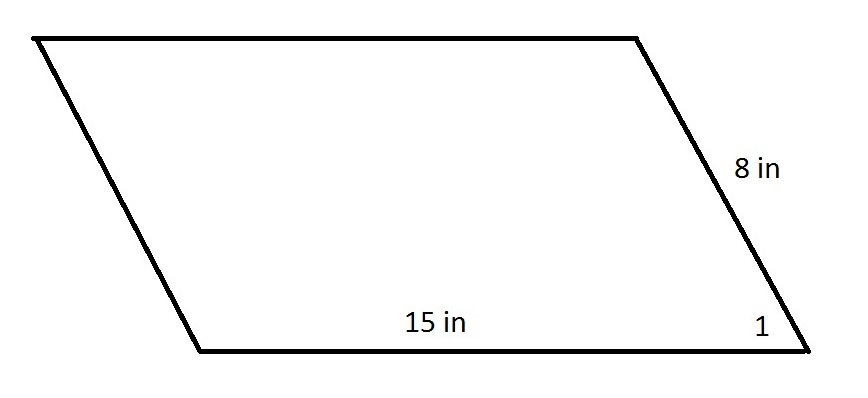In the above parallelogram,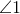is acute. Which is the greater quantity?

(A) The area of the parallelogram

(B) 120 square inches

(A) is greater

(B) is greater

(A) and (B) are equal

It is impossible to determine which is greater from the information given

(B) is greater

Explanation:

Sinceis acute, a right triangle can be constructed with an altitude as one leg and a side as the hypotenuse, as is shown here. The height of the triangle must be less than its sidelength of 8 inches.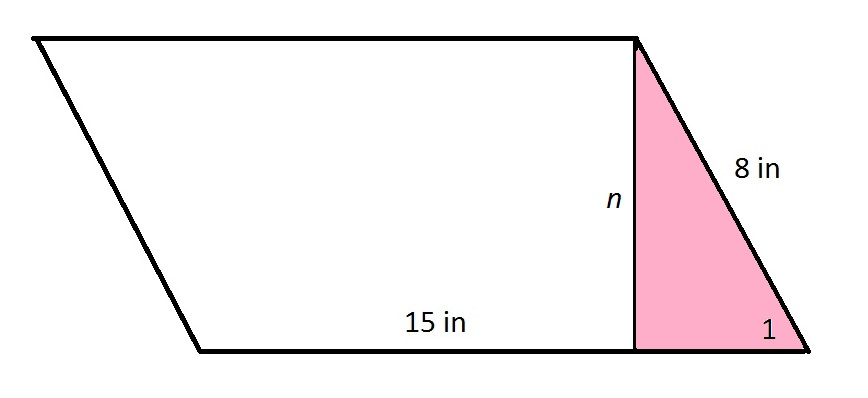The height of the parallelogram must be less than its sidelength of 8 inches.

The area of the parallelogram is the product of the base and the height - which is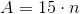Therefore,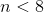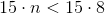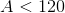(B) is greater.

### Example Question #2 : Parallelograms

Parallelogram A is below: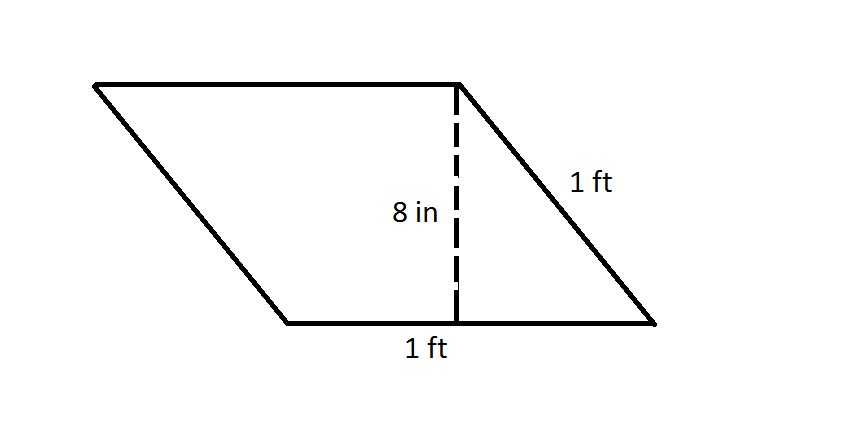Parallelogram B is below: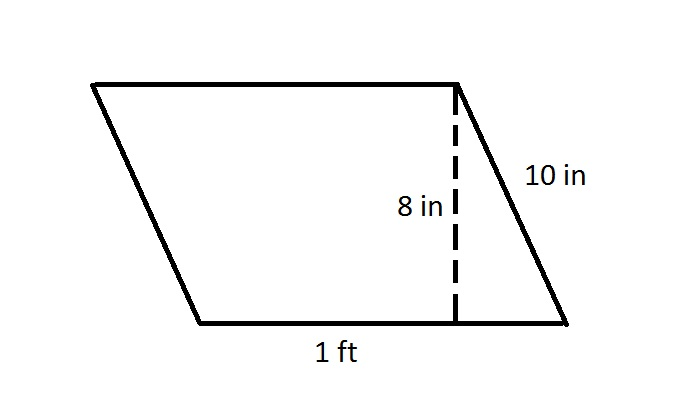Note: These figures are NOT drawn to scale.

Refer to the parallelograms above. Which is the greater quantity?

(A) The area of parallelogram A

(B) The area of parallelogram B

(A) is greater

(A) and (B) are equal

(B) is greater

It is impossible to determine which is greater from the information given

(A) and (B) are equal

Explanation:

The area of a parallelogram is the product of its height and its base; its slant length is irrelevant. Both parallelograms have the same height (8 inches) and the same base (1 foot, or 12 inches), so they have the same areas.

### Example Question #3 : Parallelograms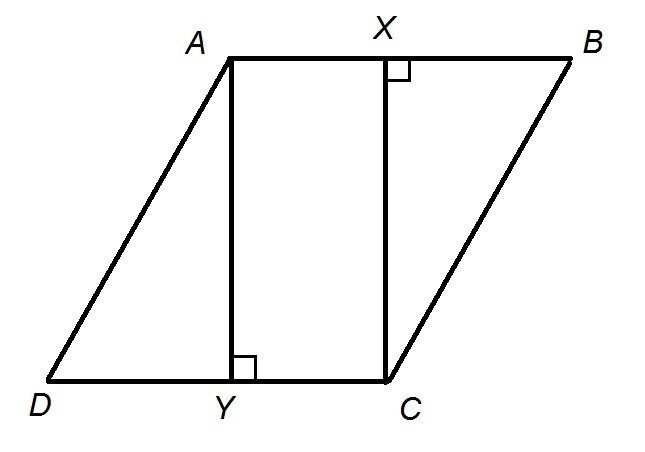Figure NOT drawn to scale

The above figure shows Rhombusand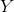are midpoints of their respective sides. Rectangle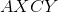has area 150.

Give the area of Rhombus.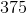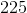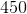Explanation:

A rhombus, by definition, has four sides of equal length. Therefore,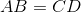. Also, sinceandare the midpoints of their respective sides,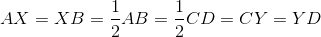We will assignto the common length of the four half-sides of the rhombus.

Also, both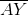and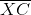are altitudes of the rhombus; the are congruent, and we will call their common length(height).

The figure, with the lengths, is below.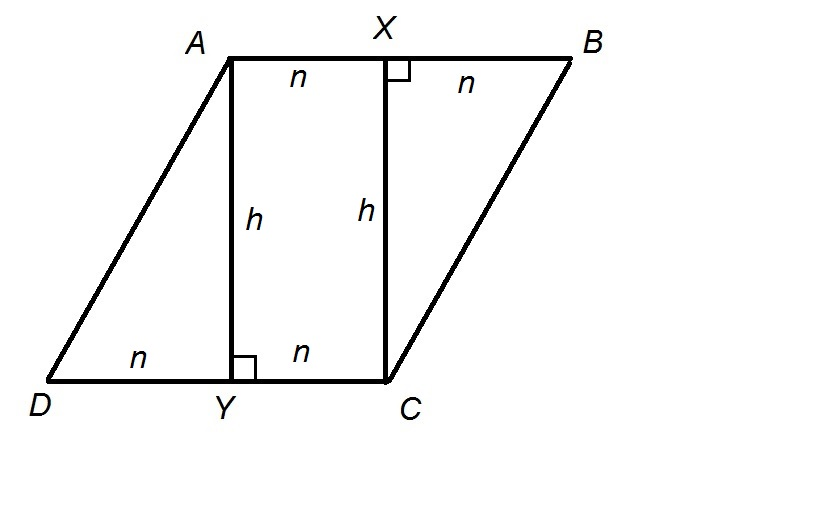Rectanglehas dimensionsand; its area, 150, is the product of these dimensions, so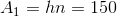The area of the entire Rhombusis the product of its heightand the length of a base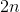, so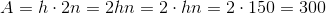.